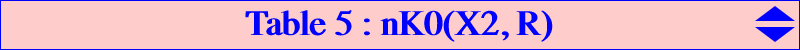catalogue name R circular nodal K+ K60 K016 Tucker+ X(2) YES K093 isotomic equilateral nK0 X(7757) YES K149 X(6) K185 Hirst-Kiepert X(523) YES K197 isotomic circular nK0 X(14568) YES K296 X(514) YESGiven a point R = u : v : w (the root of the cubic), the isotomic nK0(X2, R) has barycentric equation : ∑ u x (y^2 + z^2) = 0. It always passes through the intercepts U, V, W of the trilinear polar of R on the sidelines of ABC. The points A and U, B and V, C and W share the same tangentials A', B', C' respectively which are three collinear points on the cubic.   Circular nK0(X2, R) nK0(X2, R) is circular if and only if R = X(14568), intersection of the lines X(2)X(39) and X(30)X(98). This is the cubic K197.   Equilateral nK0(X2, R) nK0(X2, R) is equilateral if and only if R = X(7757), midpoint of X(2) and X(194). This is the cubic K093.   nK0+(X2, R) nK0(X2, R) has concurring asymptotes if and only if R lies on the cubic which is the reflection of K015 (Tucker nodal cubic) in G. In particular, with R = G, we obtain nK0+(X2, X2) = K016 = Tucker+ cubic.   Nodal nK0(X2, R) nK0(X2, R) is a nodal cubic if and only if it contains one and only one of the points G, Ga, Gb, Gc (vertices of the antimedial triangle) which is therefore the node. If we take the centroid G = X(2), R must lie on the line at infinity. When R = X(523), we obtain K185 the only nodal cubic with perpendicular nodal tangents.Points on the circumcircle (O) nK0(X2, R) meets (O) at A, B, C and three other (not always all real) points Q1, Q2, Q3. The Simson lines of these points concur (at X) if and only if R lies on the line passing through X(2), X(39), X(76), etc, in which case X lies on the line passing through X(4), X(69), X(76), X(264), etc. With R = X(2), the cubic is K016 and X = X(15030). The antipodes of Q1, Q2, Q3 on (O) lie on an isogonal pK with pivot P on the line passing through X(20), X(185). These also lie on a pK with pivot X(4) and pole Ω on the line passing through X(25), X(393). With K016, we obtain pK(X6, X15072).